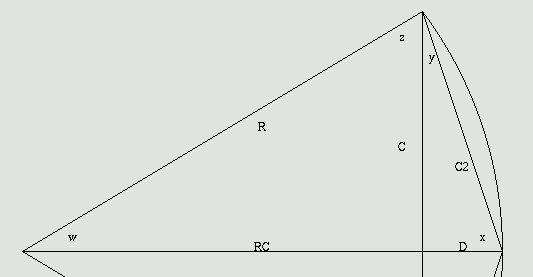```Chord :  *
End to End
End to Center

Offset:  *

```

* values like 3, 1/2, 3-3/4, 4 1/2, 7.5cm, etc. Add mm or cm for metric.C is half the distance from end to end. D is the offset amount of the curve.

```RC+D = R
RC = R-D
RC^2 + C^2 = R^2
(R-D)^2 + C^2 = R^2
R^2 - 2*R*D + D^2 + C^2 = R^2
D^2 + C^2 = 2*R*D
R = (D^2 + C^2) / (2*D)
```
See the CGI source